Normal Distribution

Set up

Normal distribution

Mathematica Summary

Normal distribution probabilities from z-scores.

In:=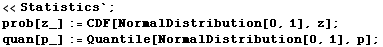Table D-4 in the text says that the probability for z ≤ -2.58 is 0.0049.  You get those numbers with these functions like this:

In:=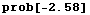Out=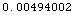In:=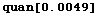Out=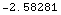Using the Error Function (Erf) Instead

Excel, TI-83

Created by Mathematica  (October 2, 2003)